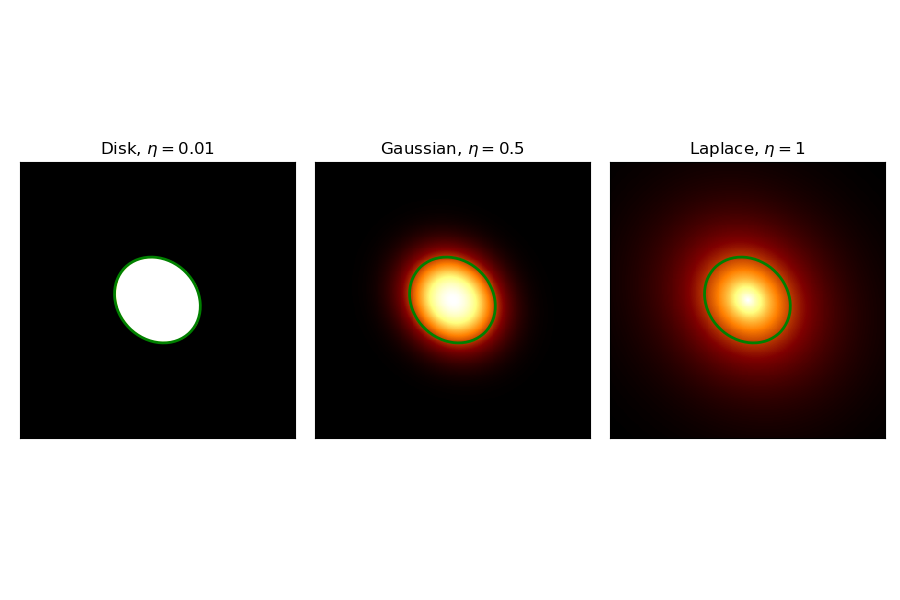# Generalized gaussian spatial model#

This is a spatial model parametrising a generalized Gaussian function.

By default, the Generalized Gaussian is defined as :

$\phi(\text{lon}, \text{lat}) = \phi(\text{r}) = N \times \exp \left[ - \left( \frac{r}{r_{\rm eff}} \right)^ \left( 1/\eta \right) \right] \,,$

the normalization is expressed as:

$N = \frac{1}{ 2 \pi \sqrt(1-e^2) r_{0}^2 \eta \Gamma(2\eta)}\,$

where $$\Gamma$$ is the gamma function. This analytical norm is approximated so it may not integrate to unity in extreme cases if ellipticity tend to one and radius is large or $$\eta$$ much larger than one (outside the default range).

The effective radius is given by:

$\r_{rm eff}(\text{lon}, \text{lat}) = \sqrt{ (r_M \sin(\Delta \phi))^2 + (r_m \cos(\Delta \phi))^2 }.$

where $$r_M$$ ($$r_m$$) is the major (minor) semiaxis, and $$\Delta \phi$$ is the difference between phi, the position angle of the model, and the position angle of the evaluation point. If the eccentricity ($$e$$) is null it reduces to $$r_0$$.

## Example plot#

Here is an example plot of the model for different shape parameter:

from astropy import units as u
import matplotlib.pyplot as plt
from gammapy.maps import Map, WcsGeom
from gammapy.modeling.models import (
GeneralizedGaussianSpatialModel,
Models,
PowerLawSpectralModel,
SkyModel,
)

lon_0 = 20
lat_0 = 0
reval = 3
dr = 0.02
geom = WcsGeom.create(
skydir=(lon_0, lat_0),
binsz=dr,
width=(2 * reval, 2 * reval),
frame="galactic",
)

tags = [r"Disk, $\eta=0.01$", r"Gaussian, $\eta=0.5$", r"Laplace, $\eta=1$"]
eta_range = [0.01, 0.5, 1]
r_0 = 1
e = 0.5
phi = 45 * u.deg
fig, axes = plt.subplots(1, 3, figsize=(9, 6))
for ax, eta, tag in zip(axes, eta_range, tags):
model = GeneralizedGaussianSpatialModel(
lon_0=lon_0 * u.deg,
lat_0=lat_0 * u.deg,
eta=eta,
r_0=r_0 * u.deg,
e=e,
phi=phi,
frame="galactic",
)
meval = model.evaluate_geom(geom)
Map.from_geom(geom=geom, data=meval.value, unit=meval.unit).plot(ax=ax)
pixreg = model.to_region().to_pixel(geom.wcs)
pixreg.plot(ax=ax, edgecolor="g", facecolor="none", lw=2)
ax.set_title(tag)
ax.set_xticks([])
ax.set_yticks([])
plt.tight_layout()## YAML representation#

Here is an example YAML file using the model:

pwl = PowerLawSpectralModel()
gengauss = GeneralizedGaussianSpatialModel()

model = SkyModel(spectral_model=pwl, spatial_model=gengauss, name="pwl-gengauss-model")
models = Models([model])

print(models.to_yaml())


Out:

components:
-   name: pwl-gengauss-model
type: SkyModel
spectral:
type: PowerLawSpectralModel
parameters:
-   name: index
value: 2.0
-   name: amplitude
value: 1.0e-12
unit: cm-2 s-1 TeV-1
-   name: reference
value: 1.0
unit: TeV
frozen: true
spatial:
type: GeneralizedGaussianSpatialModel
frame: icrs
parameters:
-   name: lon_0
value: 0.0
unit: deg
-   name: lat_0
value: 0.0
unit: deg
-   name: r_0
value: 1.0
unit: deg
-   name: eta
value: 0.5
-   name: e
value: 0.0
frozen: true
-   name: phi
value: 0.0
unit: deg
frozen: true


Gallery generated by Sphinx-Gallery